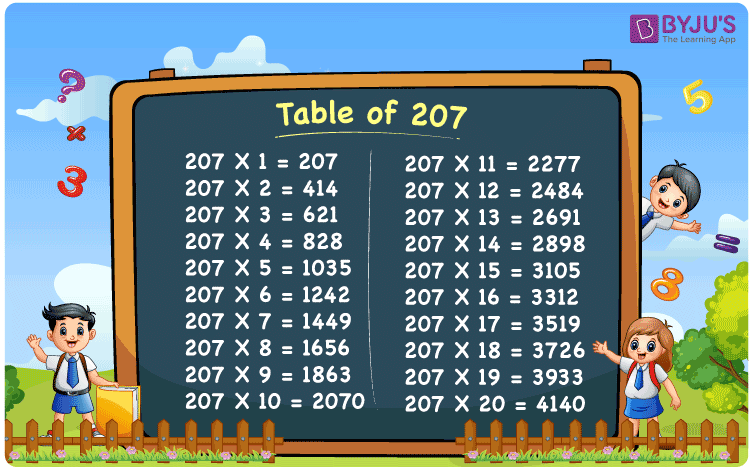Checkout JEE MAINS 2022 Question Paper Analysis : Checkout JEE MAINS 2022 Question Paper Analysis :

# Table of 207

The Multiplication Table for 207 is nothing more than the repetitive addition of the number 207. Observe the pattern in the last two digits till the multiple of 10.The numbers are from the times table of 7. And the first 2 digits are from the tables of 2. Use the Multiplication Calculator, for more multiples of 207.

## Table of 207 Chart## What is the 207 Times Table?

Any multiplication table is a repetitive addition. In the table of 207, we are repeatedly adding 207 multiple times. And the table below gives repetitive addition 10 times for the number 207.

 207 × 1 = 207 207 207 × 2 = 414 207 + 207 = 414 207 × 3 = 621 207 + 207 + 207 = 621 207 × 4 = 828 207 + 207 + 207 + 207 = 828 207 × 5 = 1035 207 + 207 + 207 + 207 + 207 = 1035 207 × 6 = 1242 207 + 207 + 207 + 207 + 207 + 207 = 1242 207 × 7 = 1449 207 + 207 + 207 + 207 + 207 + 207 + 207 = 1449 207 × 8 = 1656 207 + 207 + 207 + 207 + 207 + 207 + 207 + 207 = 1656 207 × 9 = 1863 207 + 207 + 207 + 207 + 207 + 207 + 207 + 207 + 207 = 1863 207 × 10 = 2070 207 + 207 + 207 + 207 + 207 + 207 + 207 + 207 + 207 + 207 = 2070

## Multiplication Table of 207

Given below is the multiplication table of 207 being done 20 times. The below table helps you with multiplication problems that have 207.

 207 × 1 = 207 207 × 2 = 414 207 × 3 = 621 207 × 4 = 828 207 × 5 = 1035 207 × 6 = 1242 207 × 7 = 1449 207 × 8 = 1656 207 × 9 = 1863 207 × 10 = 2070 207 × 11 = 2277 207 × 12 = 2484 207 × 13 = 2691 207 × 14 = 2898 207 × 15 = 3105 207 × 16 = 3312 207 × 17 = 3519 207 × 18 = 3726 207 × 19 = 3933 207 × 20 = 4140

## Solved Example on Table of 207

Example:

Evaluate 207 x  22 ?

Solution: 4554.

The above problem can be written as 207 x 20 + 207 x 2

= 4140 + 414 (Refer the table above)

= 4554.

## Frequently Asked Questions on Table of 207

### Question 1: Are 2 and 7 factors for 207?

Answer: No. 1, 3, 9, 23, 69 and 207 are factors for 207.

### Question 2: When do we get 3933 in the table of 207?

Answer: 19 times 207, we get 3933.

### Question 3: What is 18 times 207?

Answer: When 207 is multiplied by 18, we get 3726.